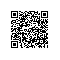trec06c
│
└───data
│   │   000
│   │   001
│   │   ...
│   └───215
└───delay
│   │   index
└───full
│   │   index


# -*- coding: utf-8 -*-
#Created by huxiaoman 2018.1.28
#transfer.py:生成spam和ham数据
import jieba
import sys
import os
import re

# 判断邮件中的字符是否是中文
def check_contain_chinese(check_str):
for ch in check_str.decode('utf-8'):
if u'\u4e00' <= ch <= u'\u9fff':
return True
return False

# 加载邮件数据的label
label_dict ={}
list1 = line.strip().split("..")
label_dict[list1.strip()] = list1.strip()
return label_dict

# 加载停用词词表
stop_dict = {}
line = line.strip()
stop_dict[line] = 1
return stop_dict

# 读取邮件数据，并转换为utf-8格式，生成spam和ham样本
parents = os.listdir(file_path)
spam_file = open(spam_file_path,'a')
ham_file = open(ham_file_path,'a')
for parent in parents:
child = os.path.join(file_path,parent)
if os.path.isdir(child):
else:
print child[10:]
label = "unk"
if child[10:] in label_dict:
label = label_dict[child[10:]]
# deal file
temp_list = []
line = line.strip().decode("gbk",'ignore').encode('utf-8')
if not check_contain_chinese(line):
continue
seg_list = jieba.cut(line, cut_all=False)
for word in seg_list:
if word in stop_dict:
continue
else:
temp_list.append(word)
line = " ".join(temp_list)
print label
if label == "spam":
spam_file.write(line.encode("utf-8","ignore") + "\n")
if label == "ham":
ham_file.write(line.encode("utf-8","ignore")+"\n")

# 生成word2vec词表
def generate_word2vec(file_path,label_dict,stop_dict,word_vec):
parents = os.listdir(file_path)
fh1 = open(word_vec,'a')
i = 0

for parent in parents:
child = os.path.join(file_path,parent)
if os.path.isdir(child):
generate_word2vec(child,label_dict,stop_dict,word_vec)
else:
print child[10:]
i += 1
print i
label = "unk"
if child[10:] in label_dict:
label = label_dict[child[10:]]
# deal file
temp_list = []
line = line.strip().decode("gbk",'ignore').encode('utf-8')
if not check_contain_chinese(line):
continue
if len(line) == 0:
continue
seg_list = jieba.cut(line, cut_all=False)
for word in seg_list:
if word in stop_dict:
continue
else:
temp_list.append(word)
line = " ".join(temp_list)
fh1.write(line.encode("utf-8","ingore")+"\n")

if __name__=="__main__":
file_path = sys.argv
label_path = sys.argv
stop_word_path = "stop_words.txt"
word_vec_path = "word2vec.txt"
spam_data = "spam.txt"
ham_data = "ham.txt"


run.sh：

bashif [ \$1 = "test" ]; then
echo "test"
python transfer.py ../test/ ../trec06c/full/index
else
echo "whole"
python transfer.py ../trec06c/data/ ../trec06c/full/index
fi


sh run.sh


ham.txt: 正样本，正常邮件。共21373条数据。

spam.txt: 负样本，垃圾邮件。共41627条数据。

word2vec.txt: 包含所有邮件分词的内容，为Word2Vec提供训练预料。共63000条数据。

Word2Vec方法的优势

# -*- coding: utf-8 -*-
# Created by huxiaoman 2018.1.28
# word2vec.py:生成word2vec模型

import os
import sys
import numpy as np
from gensim.models.word2vec import Word2Vec
from gensim.corpora.dictionary import Dictionary
import codecs

sys.setdefaultencoding( "utf-8" )

class MySentences(object):
def __init__(self, dirname):
self.dirname = dirname

def __iter__(self):
for fname in os.listdir(self.dirname):
for line in codecs.open(os.path.join(self.dirname, fname),"r", encoding="utf-8",errors="ignore"):
yield line.strip().split()

# word2vec.txt数据的地址
train_path = "rawData/"

# 生成的word2vec模型的地址
model_path = "/modelPath/"
sentences = MySentences(train_path)

# 此处min_count=5代表5元模型，size=100代表词向量维度，worker=15表示15个线程
model = Word2Vec(sentences,min_count = 5,size=100,workers=15)

#保存模型
model.save(model_path+'/Word2vec_model.pkl')


python word2vec.py


Word2vec_model.pkl


# 构建svm模型，加载数据等代码详见github
def get_svm_model(x_train,y_train,x_val,y_val):
model = SVC(C=1,kernel='rbf',max_iter=10,gamma=1,probability=True)
model.fit(x_train,y_train)
pred=model.predict(x_val)
fpr,tpr,thresholds = roc_curve(y_val, pred, pos_label=2)
score = metrics.f1_score(y_val,pred)
print score


python train_svm.py


0.73343221Python爱好者使用钉钉扫一扫加入圈子
+ 订阅